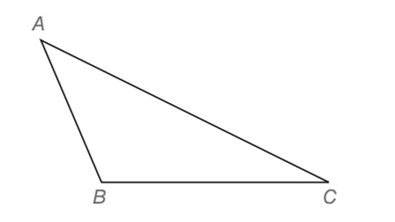Chapter 7.1, Problem 4E### Elementary Geometry for College St...

6th Edition
Daniel C. Alexander + 1 other
ISBN: 9781285195698

#### Solutions

Chapter
Section### Elementary Geometry for College St...

6th Edition
Daniel C. Alexander + 1 other
ISBN: 9781285195698
Textbook Problem
1 views

# In Exercises 3 to 8, use the drawing provided.Given: Obtuse ∆ A B C Construct: The bisector of ∠ B A C

To determine

To construct:

The bisector of BAC for the obtuse ABC as shown in the given figure.

Explanation

Definition:

The locus of points in a plane and equidistant from the sides of an angle is the angle bisector.

Calculation:

The given obtuse ABC is shown below:

To construct a bisector we follow the below procedure.

Step 1: Using the compass, take a length shorter than AB and AC and take A as centre, draw an arc on the sides of AB and AC and label them as 1 and 2 respectively.

Step 2: Take 1 and 2 as centre and draw the arcs inside the triangle to obtain a point D

### Still sussing out bartleby?

Check out a sample textbook solution.

See a sample solution

#### The Solution to Your Study Problems

Bartleby provides explanations to thousands of textbook problems written by our experts, many with advanced degrees!

Get Started

#### Define (a) a polynomial function and (b) a rational function. Give an example of each.

Applied Calculus for the Managerial, Life, and Social Sciences: A Brief Approach

#### Evaluate the indefinite integral. e5rdr

Single Variable Calculus: Early Transcendentals, Volume I

#### What is the lowest score in the following distribution?

Essentials of Statistics for The Behavioral Sciences (MindTap Course List)

#### For f(x)=exx,f(x)= a) ex b) xex1x2 c) exxexx2 d) xexxx2

Study Guide for Stewart's Single Variable Calculus: Early Transcendentals, 8th